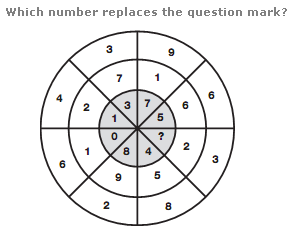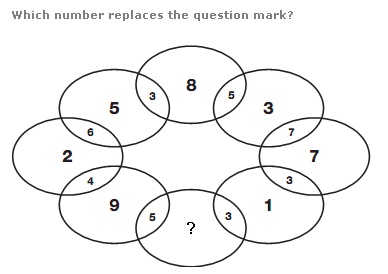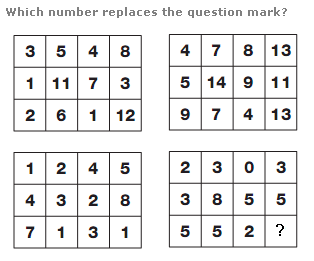# Puzzles - Number puzzles

### Exercise :: Number puzzlesAnswer : 2 Explanation : In each slice of the circle, the difference between the outer and middle numbers is written in the inner segment of the opposite slice.Answer : 4 Explanation : Calculate the difference between adjacent numbers in the centre of each oval and put the result in the space between the two ovals in the opposite side of the diagram.Answer : 11 Explanation : Numbers in the top right hand grid equal the sum of the numbers in corresponding positions in the upper and lower left hand grids, and numbers in the lower right hand grid equal the difference between numbers in corresponding positions in the upper and lower left hand grids.